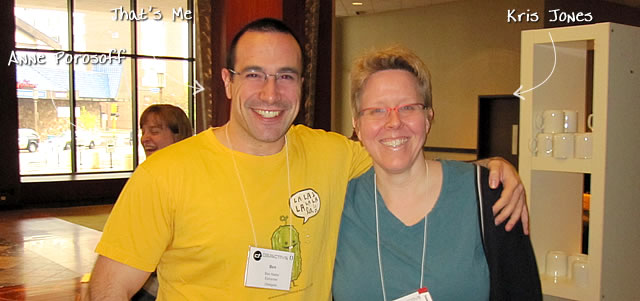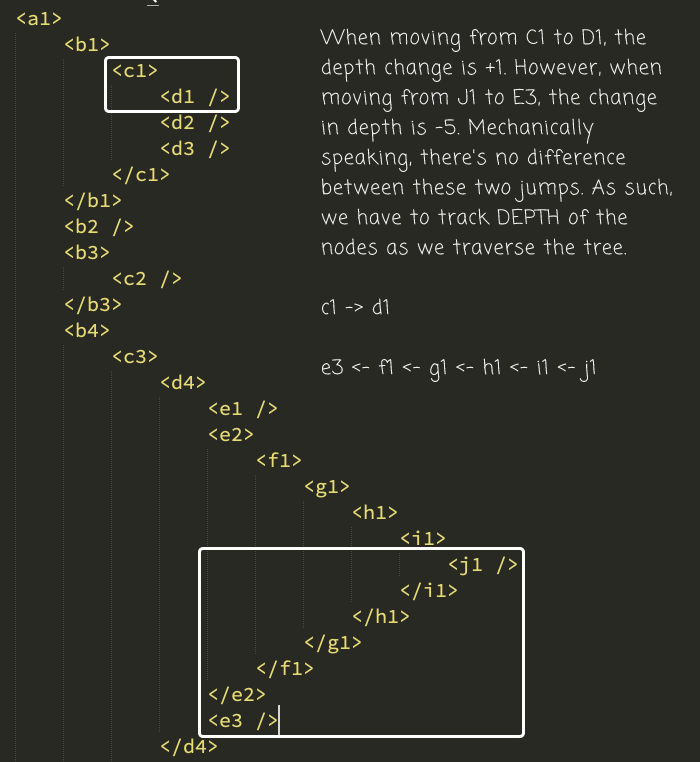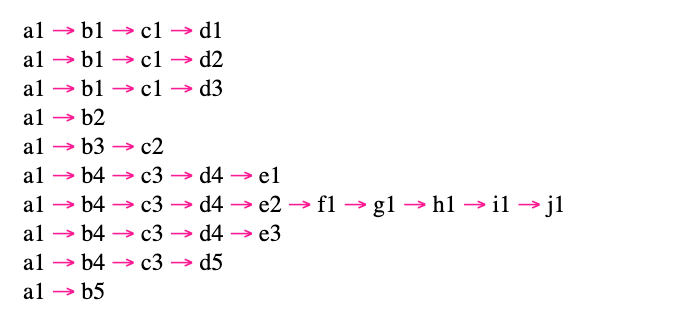Ben Nadel at cf.Objective() 2010 (Minneapolis, MN) with: Kris Jones and Anne Porosoff ( @AnnePorosoff )

# Finding All Unique Paths In A Tree Structure In Lucee CFML 5.3.7.47

By on
Tags:

At InVision, I've been working with Holger Schueler to generate some internal reports regarding how different users end up in an enterprise context. One of the reports creates a Tree structure of cascading "invitations" (ie, this user invited another user who, in turn, invited three more users). In addition to rendering the tree structure visually, Holger would also like to download the tree structure as a CSV (Comma-Separated Values) file in which each row in the CSV contains a distinct path within the tree structure. This is a data translation that I've never actually done before. So, I wanted to sit down and figure it out in Lucee CFML 5.3.7.47.

A year ago, I looked at using `while` loops to perform depth-first and breadth-first traversal of a tree structure in Lucee CFML. To find the unique paths within a tree, I'm going to use the same traversal technique; except, in addition to walking the tree, I'm also going to be aggregating the nodes into paths.

Once you understand how to use a `while` loop to perform the tree traversal, the complicated part becomes understanding how to manipulate the aggregate path as you move from node-to-node. For example, one node might be at depth `N`; then, the next node could be at depth `N+1` or something radically different like depth `N-10`. It all depends on the structure of the tree:To deal with the erratic depth changes, we have to store the depth of each node as we're performing the tree traversal. This way, as we reach each node, we can use its depth to readjust the current path aggregate. For example, imagine that we are at the current path:

`[ a, b, c, d, e, f, g ]`

... then, our next node - `N` - is at depth 3. What we need to do is trim the path aggregate down to `depth - 1` (or, 3 - 1 = 2)

`[ a, b ]`

... such that we can then append the new node at the target depth, 3:

`[ a, b, N ]`

Once I figured this approach out, all I had to do was update my queuing technique to store a "node location" rather than just a node. This location would contain the depth and the node in a single structure.

Here's the algorithm I finally came up with. To explore this concept, I'm using an XML data structure since it's easy to understand visually and make creating the sample tree data very easy:

``````<cfscript>

// Setup our Tree for demonstration purposes. Each node in this tree is named based
// on its depth and its order at a particular depth.
tree = xmlParse("
<a1>
<b1>
<c1>
<d1 />
<d2 />
<d3 />
</c1>
</b1>
<b2 />
<b3>
<c2 />
</b3>
<b4>
<c3>
<d4>
<e1 />
<e2>
<f1>
<g1>
<h1>
<i1>
<j1 />
</i1>
</h1>
</g1>
</f1>
</e2>
<e3 />
</d4>
<d5 />
</c3>
</b4>
<b5 />
</a1>
");

printTreePaths( tree );

// ------------------------------------------------------------------------------- //
// ------------------------------------------------------------------------------- //

/**
* I perform a depth-first traversal of the given XML document, printing out the set
* of unique paths to every leaf-node.
*
* @tree I am the XML Document being walked.
*/
public void function printTreePaths( required xml tree ) {

// The "recursive" nature of this tree traversal will be powered by this queue so
// that we don't have to create a deep recursive call-stack. As each node is
// visited, its child-nodes will be PREPENDED to the queue for further traversal.
// Each node will be stored along with its depth so that we know how to "reset"
// the path as we move from node to node.
// --
// ASIDE: "Prepending" nodes creates a depth-first traversal while "Appending"
// nodes creates a breadth-first traversal.
var queue = [{
node: tree.xmlRoot,
depth: 1
}];
var paths = [];
var path = [];

// Continue pulling nodes OFF THE FRONT of the queue until we have visited the
// entire tree (depth-first).
while ( queue.isDefined( 1 ) ) {

var queueItem = arrayShift( queue );
var node = queueItem.node;
var depth = queueItem.depth;

// As we move to each node, we may be moving BACK UP in the tree depth. The
// size of this jump in depth will change based on the tree structure. As
// such, we have to "trim" the current path back to what it was ABOVE THIS
// NODE so that we then add this next as the LAST ITEM in the current path.
arrayTrimTo( path, ( depth - 1 ) )
.append( node )
;

// If there are any child nodes, queue them up for further exploration.
if ( node.xmlChildren.len() ) {

node.xmlChildren.each(
( childNode, i ) => {

// NOTE: All child-nodes are at the same depth in the tree.
var newQueueItem = {
node: childNode,
depth: ( depth + 1 )
};

queue.insertAt( i, newQueueItem );

}
);

// If this node has no children, it is a LEAF NODE; which means, it's the
// terminal node in the current path. As such, we can store a copy of the
// current path into the set of all collected paths.
} else {

paths.append( path.slice( 1 ) );

}

}

// At this point, we've collected all of the paths in the tree structure. But,
// that paths consist of complex XML data-structures. We need to map them onto
// simple node-name representations.
var delimiter = " <span style='color: deeppink ;'>&rarr;</span> "

for ( var path in paths ) {

var stringifiedPath = arrayPluck( path, "xmlName" )
.toList( delimiter )
;

echo( stringifiedPath & "<br />" );

}

}

// ------------------------------------------------------------------------------- //
// ------------------------------------------------------------------------------- //

// CAUTION: The following array functions may MUTATE the original array, which only
// "works" as expected in Lucee CFML because arrays are PASSED BY REFERENCE. If your
// runtime passes arrays by VALUE, none of this will work.

public array function arrayPluck(
required array values,
required string propertyName
) {

var propertyValues = values.map(
( value ) => {

return( value[ propertyName ] );

}
);

return( propertyValues );

}

/**
* I remove the first value in the array and return it.
*
* @values I am the array being mutated.
*/
public any function arrayShift( required array values ) {

var firstValue = values.first();
values.deleteAt( 1 );

return( firstValue );

}

/**
* I reduce the size of the array until it is less-than-or-equal-to the given length.
*
* @values I am the array being mutated.
* @length I am the length to which the array is being trimmed.
*/
public array function arrayTrimTo(
required array values,
required numeric length
) {

for ( var i = values.len() ; i > length ; i-- ) {

values.deleteAt( i );

}

return( values );

}

</cfscript>
``````

And, when we run this depth-first, path-aggregation algorithm in Lucee CFML, we get the following page output:As you can see, each unique branch path in the tree has been represented as an individual line in this output; with, common ancestor nodes being repeated as necessary. And, now I know one possible way to represent a tree structure as a CSV file.

These kind of explorations are fun - so much of my work is just CRUD (Create, Read, Update, Delete), it's nice to have to think about something that requires a different part of my brain. And, I love how simple so much of this is thanks to Lucee CFML.

Want to use code from this post? Check out the license.It's amazing how I can read and understand Lucee to an extend even when I don't know anything about it.

It's also interesting that Lucee Array starts at 1@Akuma,

Sir, you don't even know how much your comment means to me :D I am so thrilled to hear that Lucee CFML is easy to ready. I totally agree; but, I've been doing it for so long, I lose sight of what is real and what is not. So, to hear you say that, it is heart-warming.

Arrays starting a "1" takes a moment to get used to when you come from another language. But, it has some great benefits. For example, if you are finding an index within an array, you can treat "1" as a Truthy:

`if ( ! values.find( x ) ) { ... }`

Since a "not found" value will return `0`, which translated to Falsy. This is in comparison to other languages that are `0`-based and have to return a `-1` for "not found", making the equivalent statement something like:

`if ( values.indedOf( x ) === -1 ) { ... }`

Of course, there are higher-level constructs that can remove some of that friction. But, there's other things where you would normally have to `length-1` in other language that you don't have to do in ColdFusion.@Ben,

I completely agree with the Array length being the true length which also limits the need for extra subtraction and many other things that come with it.

I really appreciate the effort you add to share your experience with real-life examples as well.